## Saturday, August 22, 2020

### I need a half-tablespoon. So I have absolutely no choice but to accept complex numbers.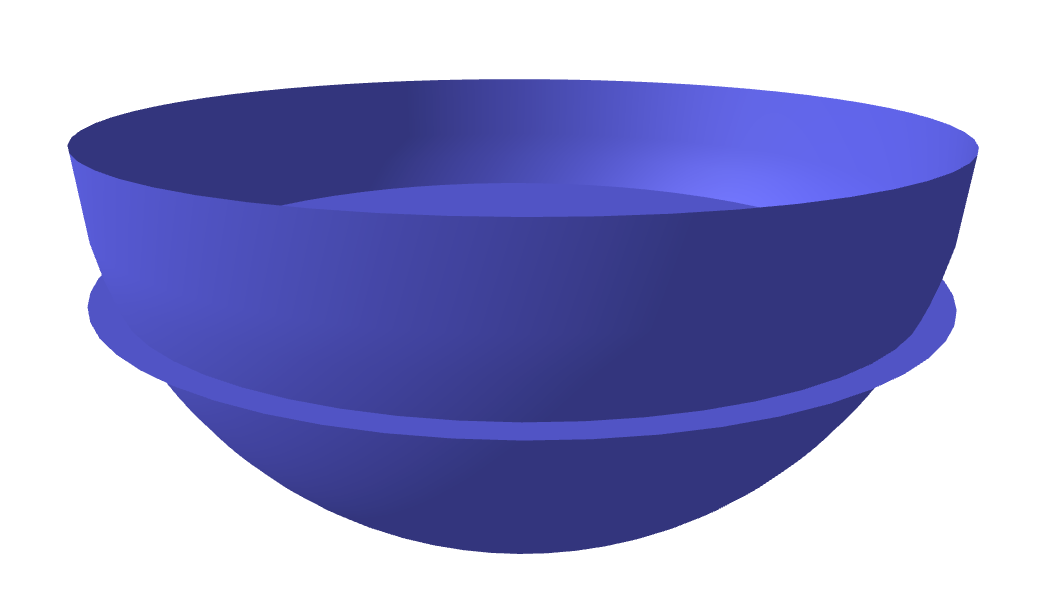You never know what math problems will show up in applications. I once had a passing thought to include the following problem on a calculus test, but I sure as heck am glad I didn't (I have included variations which seemed just slightly different from this, but solving for a different variable). Anyway so here's the story. I use measuring spoons (and cups) when I cook (I may already cause offense by doing so, lol). Notably, my measuring spoons are hemispherical. I needed a half tablespoon of something. A bit of soy sauce, being the Real Asian™ that I am. I'm missing a few teaspoon measures, due to a mishap in the garbage disposer (oops). So not having my usual measures for 1½ teaspoons (or 3/2 teaspoons, since mathematicians like improper fractions), involving very easy math, like multiplication by 3, I decided, well, why don't I just fill the hemisphere to the exact height needed to get a volume of half a hemisphere? Easy peasy. Shouldn't be much harder than multiplying by 3, right? Well... turns out to involve the number 3 in something way, way more complicated.

Now the calculus problem that I have definitely put on exams is: if you fill a hemisphere (of radius $R$) to exactly half the height ($\frac{1}{2} R$), what's the volume of such a thing? Now of course, because the curve of the hemisphere changes with the height, filling to the halfway height point is going to give you less than half the volume. Realizing the volume as a stack of disks, the answer is

$\int_{R/2}^{R} \pi(R^2 - z^2) \; dz = \frac{2\pi}{3} R^3 - \int_0^{R/2} \pi(R^2 - z^2) \; dz = \frac{2\pi}{3} R^3 - \pi R^2 z + \frac{\pi}{3} z^3 = \frac{2\pi}{3}R^3 - \frac{\pi}{2} R^3 +\frac{\pi}{3\cdot 8} R^3 = \frac{5\pi}{24} R^3.$

Oh. I thought it involved $\sqrt{2} - 1$ or something. Oh well. My memory is not what it used to be, I guess. I think that may be the answer to my volume-of-a-Sno-Cone problem, lol, which is the volume of the segment of spherical cap cut off by a cone. I don't feel like busting out the spherical coordinates today, anyway, to do that problem, lol. Some other time!

Anyway, this is of course, not half a hemisphere's worth, which is $\frac{1}{3} \pi R^3$. To get that, we have to solve for the height. It is easier to measure from the top of the spoon ($xy$-plane) than from the bottom, so we solve

$\frac13 R^3 = \int_0^h \pi(R^2 - z^2) \; dz = hR^3-\frac{1}{3} \pi h^3.$

for $h$. Simple, right? Clearing denominators and moving stuff to the other side, and canceling off the pesky $\pi$, we end up with

$h^3 - 3R^3 h + R^3 = 0$.

A nontrivial cubic polynomial. As it happens, this is the case of the depressed cubic (insert many jokes/puns here and catch flack from the sensitivity police for making light of serious, real mental illnesses). Of course, using Mathematician's Privilege (dimensional consistency is such a ... physicist ... thing), we can just set our units to $1$ and be done with it; this gives us the polynomial

$h^3 -3h + 1 = 0$.

Now I could have also tried to have my college algebra students solve this one. Rational roots theorem and all that. Nope, not gonna work, throwing $1$ and $-1$ at it. It turns out that this one is a true casus irreducibilis. It being a depressed cubic, I don't have to deal with the Germans, and can skip directly to Italians with fancy cars (perhaps I'll "retcon" my story and say I was cooking a nice risotto and needed to use half a hemispherical teacup), the solution to this is

$\sqrt{-\frac{1}{2} + \sqrt{\frac{1}{4} - 1}} + \sqrt{-\frac{1}{2} - \sqrt{\frac{1}{4} - 1}}$,

$\zeta\sqrt{-\frac{1}{2} + \sqrt{\frac{1}{4} - 1}} + \bar\zeta\sqrt{-\frac{1}{2} - \sqrt{\frac{1}{4} - 1}}$,

$\bar \zeta\sqrt{-\frac{1}{2} + \sqrt{\frac{1}{4} - 1}} + \zeta \sqrt{-\frac{1}{2} -\sqrt{\frac{1}{4} - 1}}$, where $\zeta$ is a cube root of unity (please print these formulas out onto posters and hold them up to enforce social distancing). It happens that $\zeta = -\frac{1}{2} + \frac{\sqrt{3}}{2}\mathsf i$, which is exactly what is under the cube root, so we're dealing with ninth roots of unity here! (who would have thought??). The way to see that these formulas work, generally from the depressed cubic, is basically to substitute $x = w - \frac{p}{3w}$, which results in a quadratic for $w^3$. That it's quadratic explains the nearly identical features of the stuff under the cube roots, but for a sign, and that it's $w^3$ that gets solved for is responsible for the cube roots outside, as well as mutiplying by roots of unity.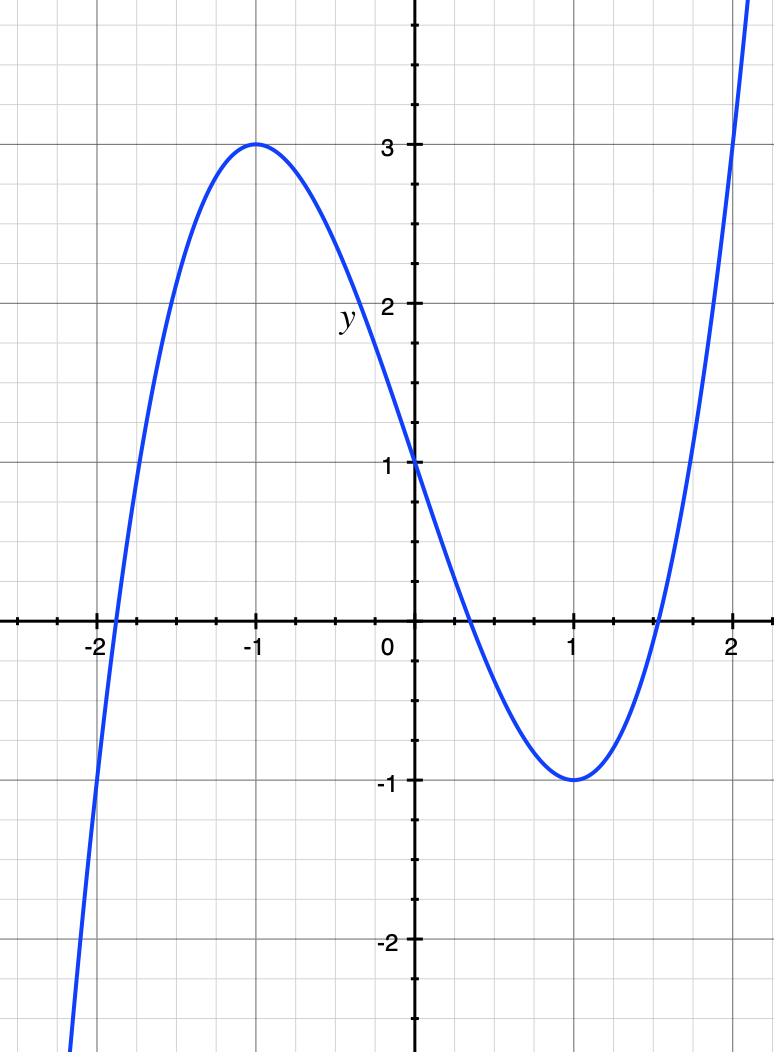The cubic $y = x^3 - 3x + 1$. It has three real roots, but they only can be expressed algebraically by the sum of complex numbers!

So the three solutions are $\sqrt{\zeta} + \sqrt{\bar{\zeta}} = \zeta^{1/3} + \zeta^{-1/3}$, $\zeta^{4/3} + \zeta^{-4/3}$, and $\zeta^{-2/3} + \zeta^{2/3}$. Taking the cube root of a complex number is not a trivial matter. It happens that the imaginary parts all cancel out and you get three real solutions, but this cannot be done without admitting complex numbers in some form (after now consulting with the French). Well, almost, anyway. Let's call in trigonometry to save the day (but that's cheating, because trigonometry really is $e^{\mathsf i x}$). We'll take $\zeta = e^{2\pi\mathsf i/3}$ and the "principal" complex cube root to take minimum argument. Actually this is irrelevant, because the extra multiplications of the $\zeta$s give you the other cube roots anyway, but for definiteness... $\zeta^{1/3}$ then is $e^{2\pi\mathsf i / 9}$ and the possible solutions are

$e^{2\pi\mathsf i / 9} + e^{-2\pi\mathsf i / 9} = 2\cos(2\pi/9)$
$e^{8\pi\mathsf i / 9} + e^{-8\pi\mathsf i / 9} = 2\cos(8\pi/9)$, and
$e^{-4\pi\mathsf i / 9} + e^{4\pi\mathsf i / 9} = 2\cos(4\pi/9)$.

Ok, so which one of these solutions is the correct one? The solution has to be between $-1$ and $1$ (in our units; the original units would be between $-R$ and $R$), and as such, only $2 \cos(4\pi/9) \approx 0.3472$ (the middle one in the graph of the full cubic above) works. So to answer the question, you need to fill up the tablespoon measure so that it is 34.72% of the way down from the top. Here's another picture and zoomed in: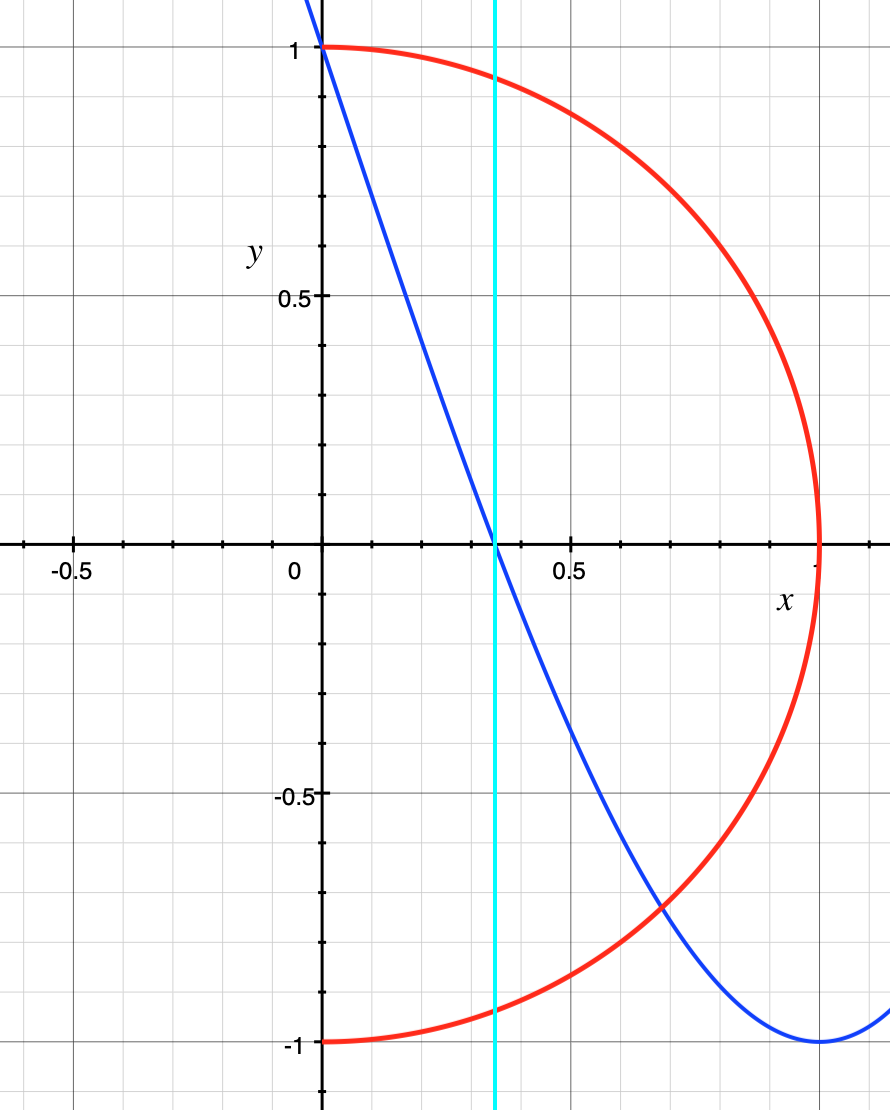A cross section of the hemisphere in question, together with a zoomed in view of the cubic curve (green). The vertical line (cyan) drawn through the cubic at its root will intersect the circle (red) where it needs to be filled (here you'd fill everything to the right of this line)

Again the fact that solving a cubic has to go through the complex numbers is why complex numbers were finally accepted. It is not, as commonly believed, due to trying to solve for the quadratic, since in that case, complex numbers only appear when the parabola fails to intersect the $x$-axis, meaning the complex solutions are of the impossible, nonexistent intersection points, so, the various Renaissance mathematicians thought, obviously should not be taken seriously. But the cubic forces it to happen no matter what, because a cubic curve always crosses the $x$-axis. So there it is: half a tablespoon? You'll need complex numbers for that. Some more views (the title image sort of is looking from above, so might obscure the fact you must fill it more than halfway from the bottom):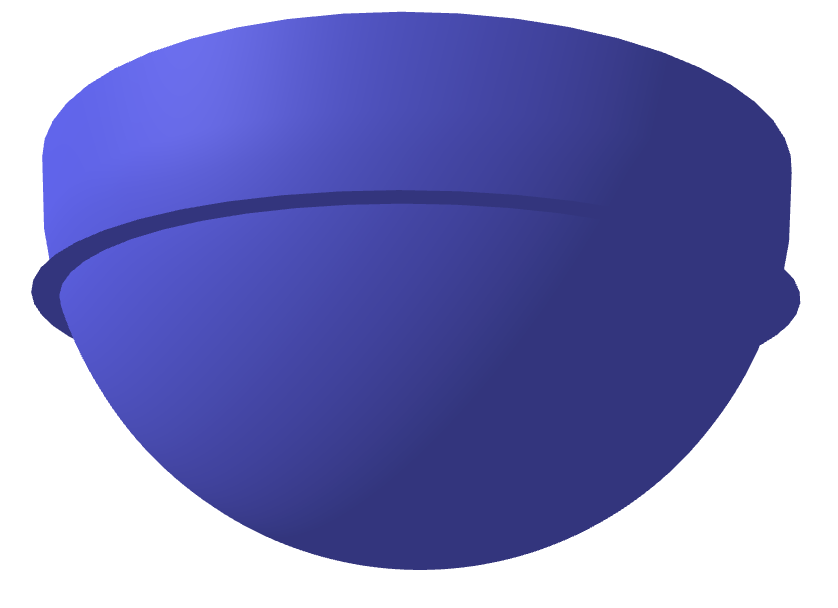View from below the tablespoon. The little extra disk sticking out is the fill line.

Finally, I'll end with more social distancing formulas: the full cubic formula $ax^3 + bx^2 + cx + d = 0$: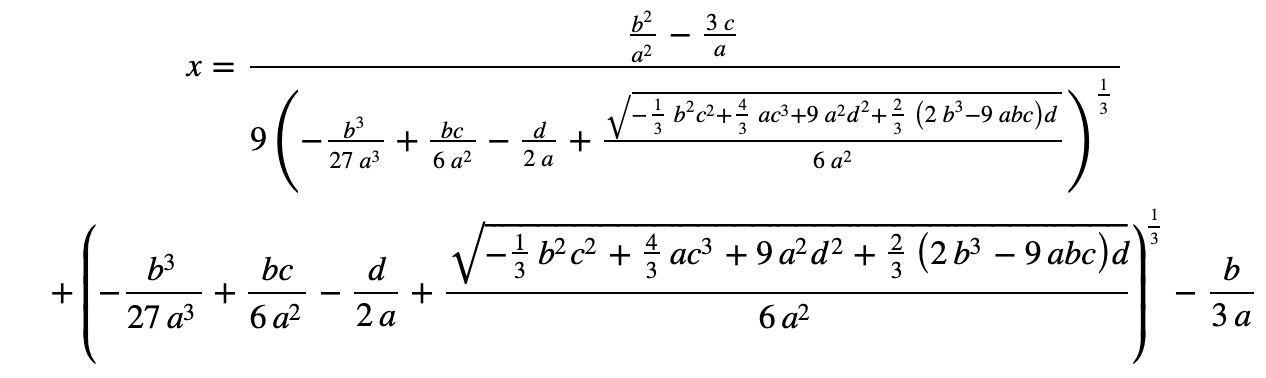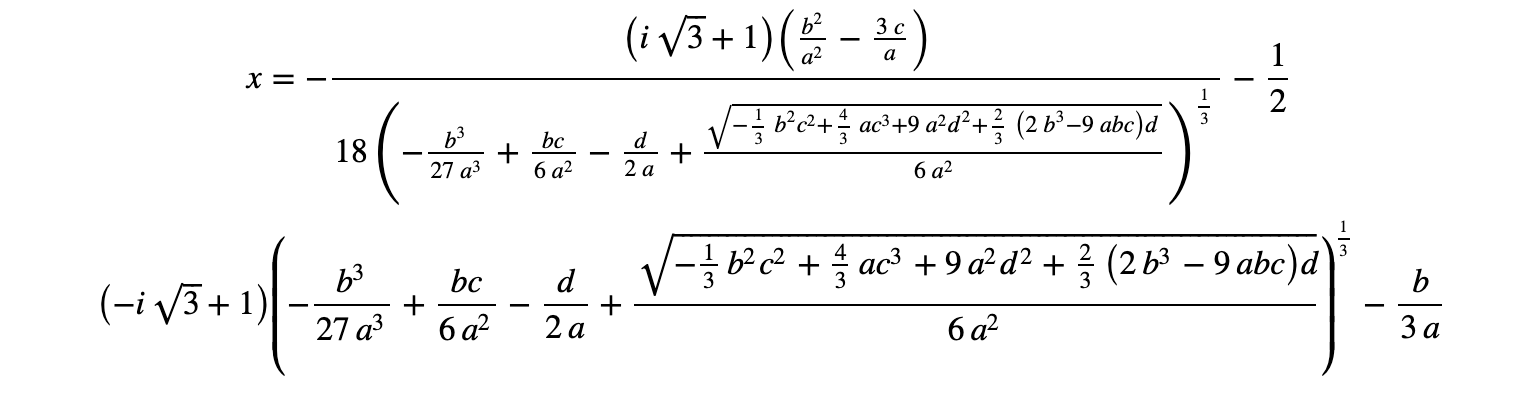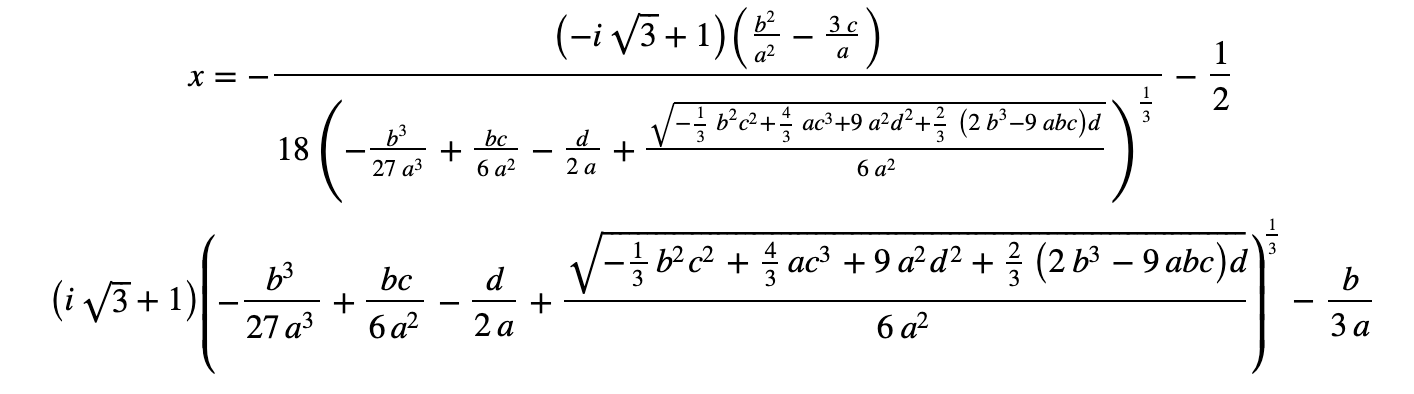For those who are interested, here's a nice video explaining this formula, and why we don't teach it in high school...

It's not taught because the solutions you get may not be easily reducible to something you can recognize, even in simple cases. Conceptually, however, it is a real adventure, and solving it by breaking it into a 2-step process makes it tractable and interesting. Our tablespoon example already skips step 1 ... all that about Germans and Italians.

Enjoy and stay safe!

1.This comment has been removed by the author.

2.This comment has been removed by the author.

3.I have to search sites with relevant information on given topic and provide them to teacher our opinion and the article. livemobile888

4.I admire this article for the well-researched content and excellent wording.I got so involved in this material that I couldn’t stop reading.I am impressed with your work and skill.Thank you so much.What Color Is Math?

5.Hi, I am Jennifer from Web Magazine and Newsin USA your blogs really help us to develop our skills, I appreciated more articles to gather knowledge.

6.Heya! I am glad to have this information, I am Lora from Web Magazine and News. This is a very helpful blog for all those who are very expressive and too much good in blogging.

7.Much of the rationale injection molding is completed in southeast Asia is price of|the value of} assembling easy components during an injection molding run. To the extent that you can design meeting out of the process you'll save vital money on price of|the value of} labor. If you have been to cut a cross-section of the Panasonic mold above you would notice that the wall thickness is roughly 2-3mm thick throughout. Keeping walls from being too thick is essential to stop inconsistencies within the cooling process resulting in defects like sink marks. After the holding part, the screw draws back, releasing strain and allowing the part to cool within the mold. This identified as|is called|is named} Direct CNC ‘cooling time’, it could possibly} additionally range from a couple of of} seconds to some minutes and ensures that the element sets appropriately before being ejected and finished on the production line.